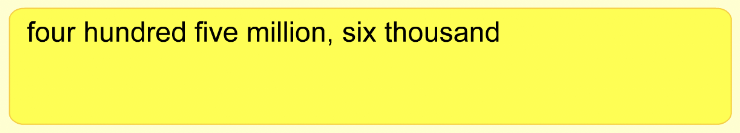Writing Large Numbers 1
Set 1 (10 Questions)
Students read and write whole numbers in the millions.
From Mr. Anker Tests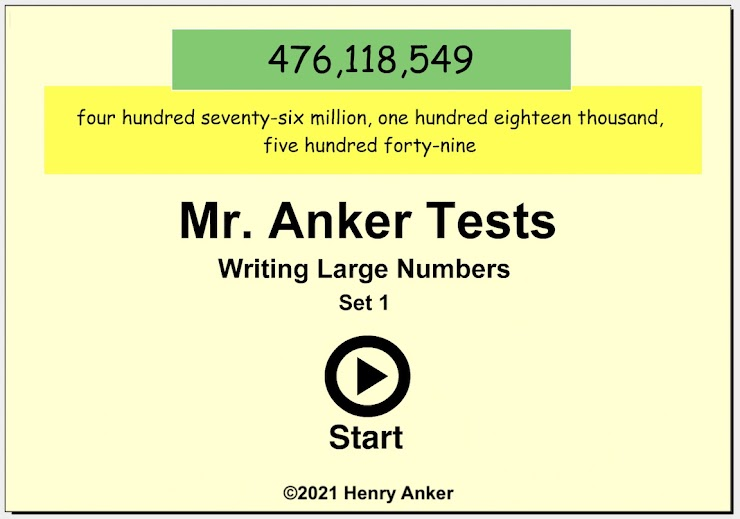1. Choose the standard form number that matches the words below. *
1 point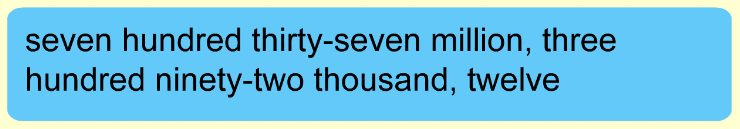*Always double-check the answer you choose, or enter by typing, before going on to the next question.
2. Choose the standard form number that matches the words below. *
1 point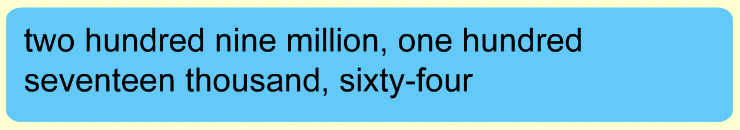3. Choose the standard form number that matches the words below. *
1 point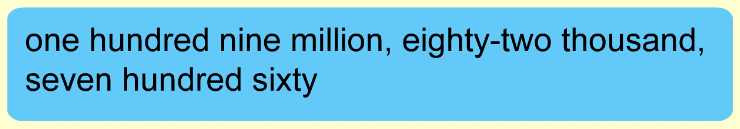4. On the line below, write the standard form number using digits and commas that matches the number as it is written out it words below. *
1 point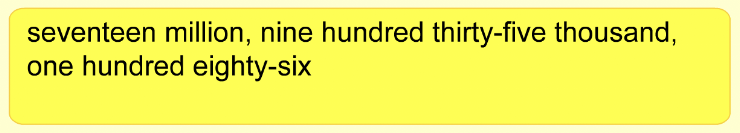5. On the line below, write the standard form number using digits and commas that matches the number as it is written out it words below. *
1 point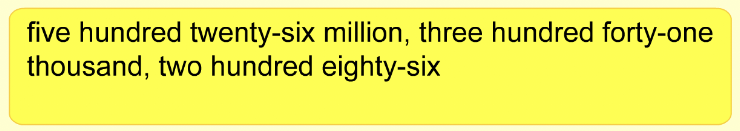6. On the line below, write the standard form number using digits and commas that matches the number as it is written out it words below. *
1 point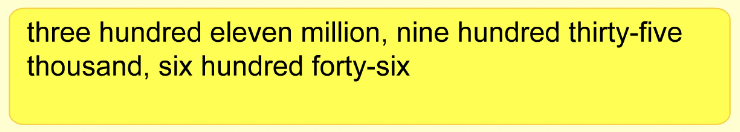7. On the line below, write the standard form number using digits and commas that matches the number as it is written out it words below. *
1 point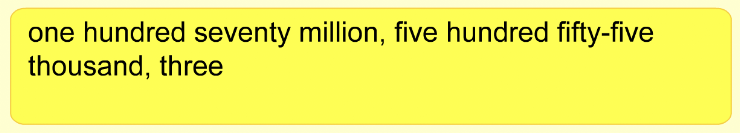8. On the line below, write the standard form number using digits and commas that matches the number as it is written out it words below. *
1 point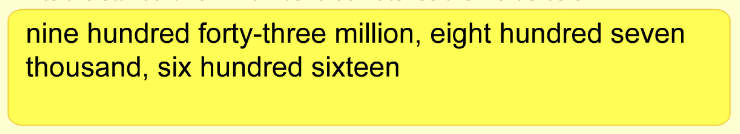9. On the line below, write the standard form number using digits and commas that matches the number as it is written out it words below. *
1 point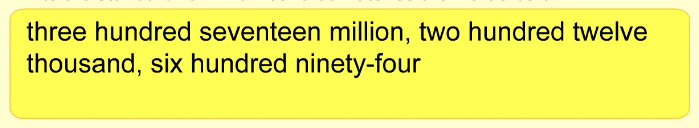10. On the line below, write the standard form number using digits and commas that matches the number as it is written out it words below. *
1 point